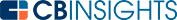# What is a Double Declining Balance?A double declining balance is an expense related to the depreciation expenses of a business. Double declining balances are used to calculate the depreciation of an asset over its useful life in a method known as the double declining balance depreciation (DDBD) method.

This type of depreciation method is known as an accelerated depreciation method since it counts more depreciation expenses upfront in the earlier years of an asset’s life.

## How to calculate double declining balance depreciation

You’ll need to take the following steps to calculate double declining balance depreciation:

• First, you need the initial cost of the asset.
• Then, the useful life of the asset needs to be determined.
• The salvage value of the asset should also be determined.
• Afterwards, the annual depreciation rate, which is the same as the straight line depreciation rate, should be determined.
• The annual depreciation rate is then doubled.
• This value is then multiplied by the book value of the asset at the beginning of the period. This is the depreciation amount for the financial period in question. In the first year, this is just the initial cost of the asset.
• The value of the asset at the end of the period is the book value at the beginning of the period minus the depreciation amount calculated above.
• To calculate the depreciation for successive years, simply repeat the steps above until the salvage value is reached. Note that the depreciation amount for the final period may need to be adjusted downwards so that the salvage value is reached.

Double declining balance depreciation is calculated using the formula below:

Double declining balance depreciation (DDBD) = 2 x annual depreciation rate (ADP) x book value (BV)

Here is a breakdown of the terms used in our formula above:

Double declining balance depreciation (DDBD): This is a method for calculating depreciation that accounts for a larger depreciation expense upfront as compared to straight line depreciation.

Annual depreciation rate (ADP): This is calculated by dividing 100% by the useful life of an asset. For example, if an asset has a useful life of 5 years, then the ADP is 100% / 5 = 20%.

Book value (BV): This is the value of an asset at the beginning of a financial period.

## Relationship to the straight line depreciation method

Both the double declining balance depreciation and straight line depreciation are commonly used to calculate depreciation.

However, while the straight line method maintains a constant level of depreciation, the DDBD method causes the depreciation to vary annually based on the changing book value. As time passes the DDBD rate drops, meaning that the depreciation expenses of an asset are reduced over time. Note that the final depreciation charge for an asset may need to be adjusted to a lower amount to ensure that the salvage value remains at the estimated amount.

## Double declining balance example

Imagine that we have a company called Linear Dynamic that purchased a vehicle for \$60,000. This vehicle is estimated to have a useful life of 5 years and a salvage value of \$5,000. The accountants at Linear Dynamic will calculate the DDBD for the vehicle using the following values.

• The initial cost of the asset is \$60,000. This means that the book value (BV) for the 1st period is \$60,000.
• The useful life of the asset is 5 years.
• The salvage value is \$5,000
• The annual depreciation rate (ADP) is 100% / 5 = 20%.

With this information at hand, the accountants are able to go ahead and calculate the DDBD for the first period using the formula discussed above:

DDBD = 2 x 20% x \$60,000 = \$24,000

After calculating the DDBD for the first year, they are able to calculate the depreciation over the vehicle’s useful life. The results are illustrated in the table below.In the final year, the DDBD is adjusted so that the salvage value is maintained at \$5,000.

## Pros and cons of double declining balance

DDBD makes sense for assets that lose value quickly such as cars, some computer equipment, and some high-tech items. In addition, for items that require more maintenance over time like cars, the bigger depreciation expense is an advantage. The bigger depreciation expenses upfront lets businesses get bigger tax write-offs in the earlier years of such assets and this can help with maintenance costs as the asset ages. The cost of the asset is also offset earlier. This can help if a loan was taken out to buy an asset since the tax write-offs can contribute towards paying off the loan earlier.

On the other hand, this method is more complicated to calculate than straight line depreciation. Businesses must also consider that as the depreciation expenses of assets decline, their tax expenses will increase.

Using the DDBD method results in larger depreciation expenses upfront. This means more tax write-offs in the early years of owning an asset. This is useful for assets that lose value quickly and can help offset the cost of assets for which money was borrowed to buy. However, this method is more complicated to calculate than straight line depreciation and as the depreciation expenses for assets go down, tax expenses go up.

You may also like# www.cbinsights.com